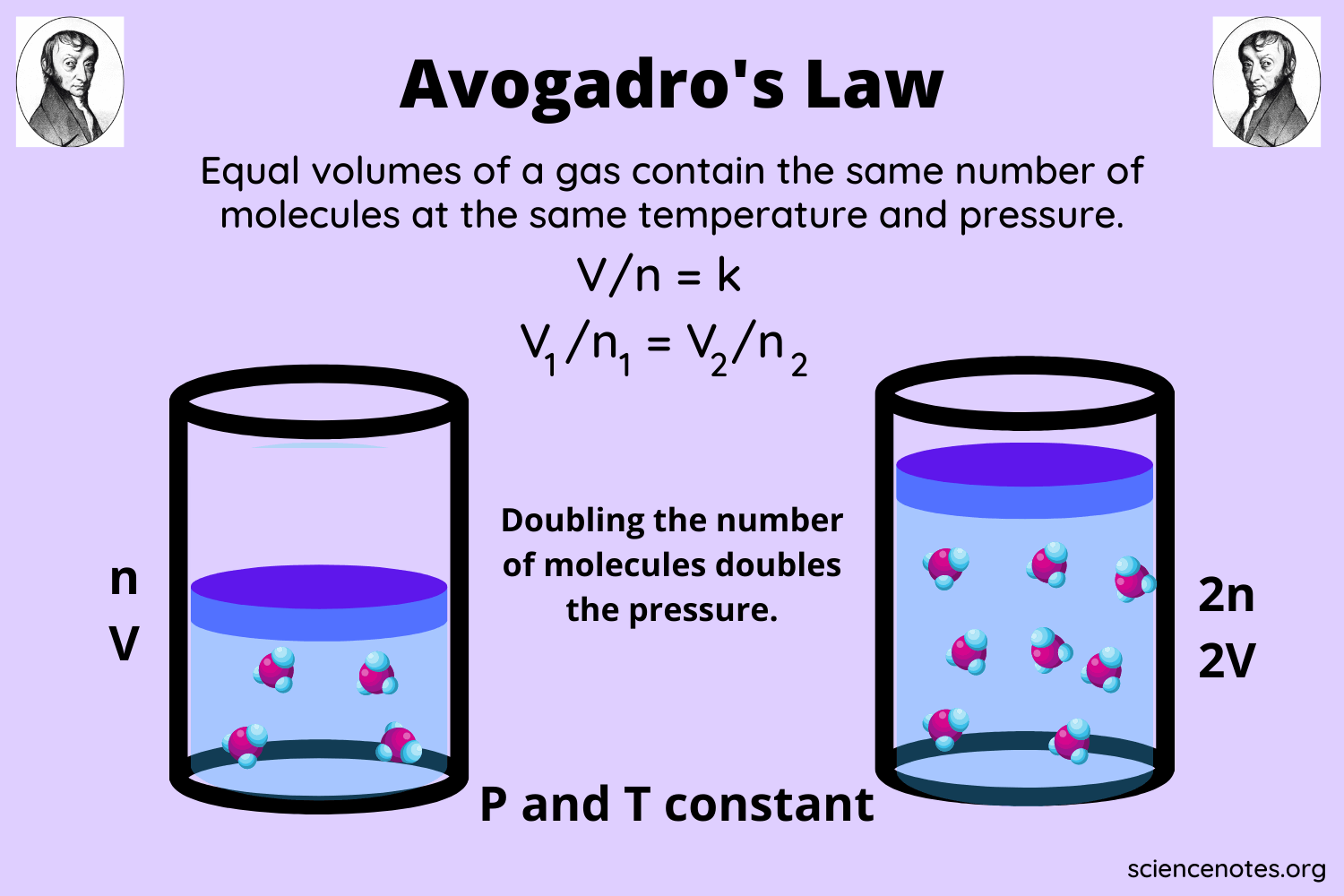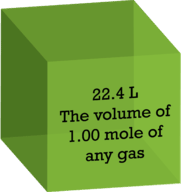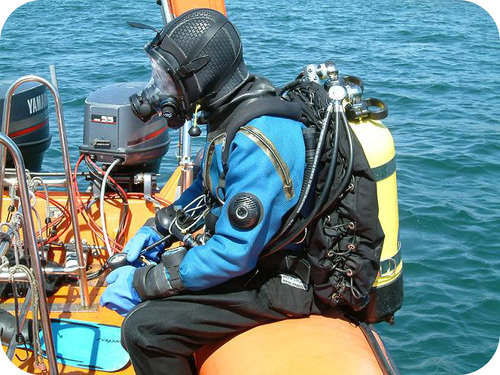INTRODUCTION

A matter exists in three states, solid, liquid, and gas. The intermolecular force in a solid is so strong that it has a high density. The particles in solid can barely move, so they occupy a lesser volume in a container. The liquid, on the other hand, has a weak intermolecular force, so the particles have some freedom of movement. Therefore, liquids occupy a greater volume in a container and assume their shape.Source

Lastly, particles in a gas have their own speed and direction, so there is a lot of empty space in between. Therefore, they occupy a higher volume in a container. However, an accurate measurement of the volume occupied by a gas is difficult. Avogadro’s hypothesis is used to determine the volume of gases. In this article, we will talk more about Avogadro’s hypothesis to provide you with a better understanding.

### WHAT IS AVOGADRO’S HYPOTHESIS DEFINITION?

Amedeo Avogadro, in 1811 proposed that different gases with the same volume have an equal number of particles under the same temperature and pressure conditions. This is known as Avogadro’s law definition. Therefore, a lighter gas like hydrogen and a heavier gas like SF₆ will have the same number of particles at equal volume.

Gas volume is affected by pressure because increasing the pressure can compress the gas particles and close the empty space between them. Hence, the volume will decrease. Similarly, heating a gas (raising the temperature) will impart kinetic energy to the particles, and they will move further apart, thereby increasing the volume.

This is the reason that Avogadro proposed that gas volume comparison must always be made under standard temperature and pressure conditions. The standard temperature is 0°C, and the standard pressure is 1 atm.

Based on these findings, it was stated that the molar volume of a gas is the volume occupied by 1 mole of gas at standard temperature and pressure conditions. Therefore, 1 mole of all the gases at STP occupy a volume of 22.4L have 6.02 X 10²³ particles.Source

The diagram above represents three gases: helium, nitrogen, and methane. All these gases contain the same number of particles at 1 mole at STP. However, each of these gases has a different molar mass. Now, is molar volume intensive or extensive? 1 mole of any gas occupies equal volume. This is an intensive property.

Scuba divers carry an oxygen tank on their back that allows them to breathe underwater. However, there is only a limited supply of oxygen, so the divers must be wary of oxygen levels to avoid any accident.

For this reason, they use different devices to take pressure readings. A basic understanding of Avogadro’s hypothesis helps them figure out the oxygen levels for scuba tank size comparison and to get back to the surface in time.Source

## CONCLUSION

• The matter exists in three states, solid, liquid, and gas.
• Measurement of matter can be done through volume, mass, and quantity.
• However, different states of matter occupy different volumes.
• Solids have a strong intermolecular force, so they have a higher density and occupy a lesser volume.
• Liquids have a weaker intermolecular force, so they occupy a greater volume in the container.
• Gases have a lot of empty space between particles because they move at their own speed and have high kinetic energy.
• Measurement of the volume of gases is difficult because of their properties.
• Avogadro’s principle definition states that the same volume of gases under standard temperature and pressure conditions contain an equal number of particles (6.02 X 10²³).
• The standard temperature is 0°C, and the standard pressure is 1 atm.
• Scuba divers apply Avogadro’s hypothesis to measure the level of oxygen left in their tank.

### FAQs

Avogadro’s constant is the number of particles contained in 1 mole of gas occupying a volume of 22.4L under standard temperature and pressure conditions. The value of Avogadro’s constant is 6.02 X 10²³.

2. What is Avogadro number mole?

The value of Avogadro’s constant is 6.02 X 10²³.

We hope you enjoyed studying this lesson and learned something cool about Avogadro’s Hypothesis! Join our Discord community to get any questions you may have answered and to engage with other students just like you! Don’t forget to download our App to experience our fun VR classrooms – we promise, it makes studying much more fun! 😎